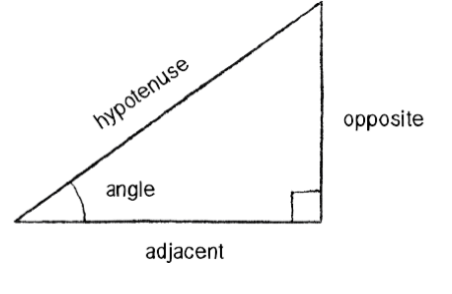# I didn't really understand this video...

• What does

$$f(x)=\sin x$$

even mean? Specifically, what is the sin function?

• @professionalbronco Welcome to the world of trigonometry!
$$\text{sin(x)}$$ is a trigonometric function. It might sound complicated, but bear with me! Hopefully you can understand the basic after reading this.

$$\text{sin(x)}$$ is acutally not alone-- the three most important trig functions are sine: $$\text{sin(x)}$$ , cosine: $$\text{cos(x)}$$, and tangent: $$\text{tan(x)}$$ (there's actually three more but don't worry about those for now).

Trig functions deal with ratios, and it's all based on triangles. That's why its called trigonometry!

Here's a helpful acronym that will help you remember which ratios each of the functions describe: SOHCAHTOA
SOH:
$$\textbf{s}\text{in(x)}=\frac{\textbf{o}\text{pposite}}{\textbf{h}\text{ypotenuse}}$$
CAH:
$$\textbf{c}\text{os(x)}=\frac{\textbf{a}\text{djacent}}{\textbf{h}\text{ypotenuse}}$$
TOA:
$$\textbf{t}\text{an(x)}=\frac{\textbf{o}\text{pposite}}{\textbf{a}\text{djacent}}$$

Now what do adjacent, opposite, and hypotenuse mean? Take a look at this diagram:One thing: when talking about these ratios, it HAS to be a right triangle. Otherwise it doesn't work the way we're doing it right now.
So, whatever the angle is, the opposite side is the side thats directly across or "opposite" from it. The hypotenuse is always the longest side in the triangle (opposite of the right angle), and the adjacent side is the third side, the one that's next to or "adjacent" to it.

Let's draw an example!In this case, the side that is opposite of the $$30^{\circ}$$ angle has length $$1$$. The adjacent side has length $$\sqrt{3}$$, and the hypotenuse has length $$2$$.

So, plug in these values into the functions and that's it! Let's do sine.
remember SOH:
$$\textbf{s}\text{in(x)}=\frac{\textbf{o}\text{pposite}}{\textbf{h}\text{ypotenuse}}$$
$$\text{sin}(30^{\circ})=\frac{1}{2}$$

And actually, this is saying that for every right triangle with a 30 degree angle, the ratio of the side opposite to the $$30^{\circ}$$ angle to the hypotenuse is ALWAYS $$\frac12$$!

You can use this process for cosine and tangent too. You can do this for any triangle too!
Hope this explains a bit• @quacker88 But still, how can I graph something like

$$y=\sin x?$$

Don't we need to specify a right triangle? I'm so confused now.

• @professionalbronco That's really great that you're thinking about triangles!
Here's the thing-- think about what $$x$$ is. It's the angle that we're looking at when trying to find the ratios.

What $$\text{sin}(30^{\circ})=\frac12$$ says is that the ratio of the side opposite of a $$30^{\circ}$$ angle in a triangle TO the hypotenuse is always equal to $$\frac12$$.

So, if you are looking at the graph of $$y=\text{sin}(x)$$, plugging in $$x=30^{\circ}$$ gives you $$y=\frac12$$. This means that $$(30,\frac12)$$ will be on the graph.

Basically, whatever you plug into $$x$$, that's the angle we're looking at. If you plug in $$x=15^{\circ}$$, we're now looking at a $$15^{\circ}$$ angle in a right triangle.

Wait-- let's go back to the point $$(30,\frac12)$$. Try doing $$\text{sin}(30)$$ on your calculator. If you get $$\frac12$$, great! But you actually might get something really weird (like -0.988).

If you do get something weird, this goes back to what the lesson was talking about, degrees vs. radians! Use what you learned in the lesson to figure out what $$30^{\circ}$$ is in radians. Then, try to take the sine of that value. That should give you $$\frac12$$.

Here's a tip to remember-- ALWAYS make sure whether you're using degrees or radians. If you're using degrees, set your calculator to degrees. Same thing with radians.

One last note: you shouldve gotten that $$30^{\circ}$$ is equal to $$\frac{\pi}{6}$$ radians. So, does that mean we should graph $$(30,\frac12)$$ or $$(\frac{\pi}{6},\frac12)?$$

Well, it depends on the graph. If the graph tells you to graph the points in degrees, use 30. If it says radians, use $$\frac{\pi}{6}$$.
But, just try to think about graphing both points. $$30$$ is pretty huge when we're graphing right? Especially compared to how small $$\frac{1}{2}$$ is. Radians, on the other hand, are much smaller. It's so much easier to find $$\frac{\pi}{6}$$ because it's smaller. So, graphs often use radians by default.